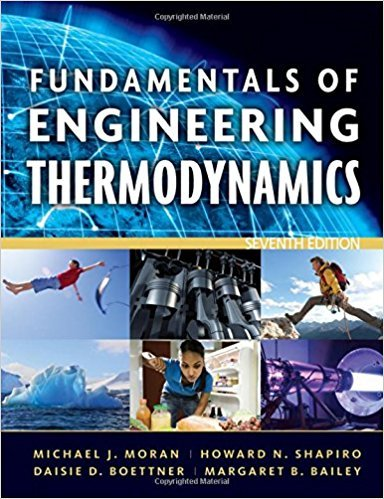×
Log in to StudySoup
Get Full Access to Fundamentals Of Engineering Thermodynamics - 7 Edition - Chapter 3 - Problem 148p
Join StudySoup for FREE
Get Full Access to Fundamentals Of Engineering Thermodynamics - 7 Edition - Chapter 3 - Problem 148p

Already have an account? Login here
×
Reset your password

# Reviewing ConceptsAnswer the following true or | Ch 3 - 148PISBN: 9780470495902 50

## Solution for problem 148P Chapter 3

Fundamentals of Engineering Thermodynamics | 7th Edition

• Textbook Solutions
• 2901 Step-by-step solutions solved by professors and subject experts
• Get 24/7 help from StudySoup virtual teaching assistantsFundamentals of Engineering Thermodynamics | 7th Edition

4 5 1 420 Reviews
18
4
Problem 148P

Problem 148P

Reviewing Concepts

Answer the following true or false. Explain

(a) The change in specific volume from saturated liquid to saturated vapor, (vg − vf), at a specified saturation pressure increases as the pressure decreases.

(b) A two-phase liquid-vapor mixture with equal volumes of saturated liquid and saturated vapor has a quality of 50%.

(c) The following assumptions apply for a liquid modeled as incompressible: The specific volume (density) is constant and the specific internal energy is a function only of temperature.

(d) Carbon dioxide (CO2) at 320 K and 55 bar can be modeled as an ideal gas.

(e) When an ideal gas undergoes a polytropic process with n = 1, the gas temperature remains constant.

Step-by-Step Solution:
Step 1 of 3

Solution 148P:

a)

This is true.

As the pressure decreases, the change in saturated liquid and saturated vapour volume decreases.

b)

This is false.

The formula to calculate the quality is:c)

This is true.

Because we know that,

if.

d)

This is true.

Carbon dioxide can be treated as an ideal gas at this temperature and pressure.

The behaviour is very similar with the ideal gas.

e)

This is true.

As we know the condition of polytropic process,When.

So, the gas temperature will not change during the process.

Step 2 of 3

Step 3 of 3

##### ISBN: 9780470495902

This full solution covers the following key subjects: saturated, liquid, vapor, specific, gas. This expansive textbook survival guide covers 14 chapters, and 1501 solutions. This textbook survival guide was created for the textbook: Fundamentals of Engineering Thermodynamics, edition: 7. Since the solution to 148P from 3 chapter was answered, more than 312 students have viewed the full step-by-step answer. The full step-by-step solution to problem: 148P from chapter: 3 was answered by , our top Engineering and Tech solution expert on 07/20/17, 09:01AM. The answer to “Reviewing ConceptsAnswer the following true or false. Explain(a) The change in specific volume from saturated liquid to saturated vapor, (vg ? vf), at a specified saturation pressure increases as the pressure decreases.________________(b) A two-phase liquid-vapor mixture with equal volumes of saturated liquid and saturated vapor has a quality of 50%.________________(c) The following assumptions apply for a liquid modeled as incompressible: The specific volume (density) is constant and the specific internal energy is a function only of temperature.________________(d) Carbon dioxide (CO2) at 320 K and 55 bar can be modeled as an ideal gas.________________(e) When an ideal gas undergoes a polytropic process with n = 1, the gas temperature remains constant.” is broken down into a number of easy to follow steps, and 110 words. Fundamentals of Engineering Thermodynamics was written by and is associated to the ISBN: 9780470495902.

Unlock Textbook Solution

Enter your email below to unlock your verified solution to:

Reviewing ConceptsAnswer the following true or | Ch 3 - 148P